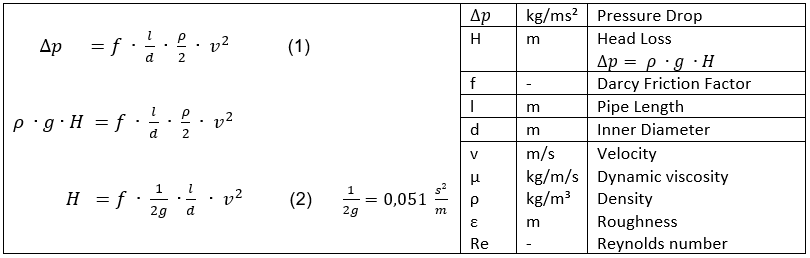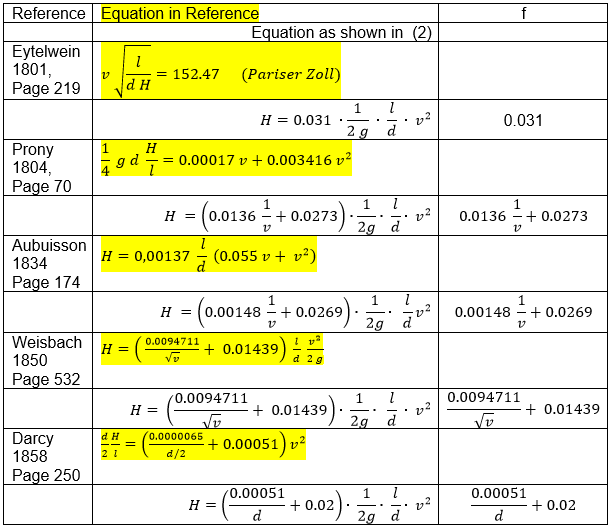﻿﻿﻿ Darcy Weisbach ﻿ ﻿ ﻿ ﻿

# L´équation de Darcy-WeisbachThe Darcy-Weisbach equation is an empirical approach for the calculation of pressure losses in straight open channels and piping. The analytical approach with a system of differential equations provided by [Navier 1822] can only be integrated for a few particular cases. Specialized software packages provide iterative solutions. In everyday life of engineers the Darcy-Weisbach equation has established itself due to easy handling.

Table 1 shows equations of major authors from the first half of the 19th century regarding pressure loss in straight piping. The empirical equations were derived from experiments with turbulent water flow in iron and cast iron pipes.Table 1

[Weisbach 1850, page 529] noted equation (1) as we know it today. Darcy Friction Factors f in Table 1 become dimensionless by the dimensions of their constants.

The approach of Eytelwein features a constant Darcy Friction Factor. Weisbach, Prony and Aubuisson show that Dracy Friction Factor f falls in value with rising velocity. Darcy points out that Friction Factor f falls in value with rising diameters. Both observations are true. The absolute Darcy Friction Factor values provided by equations of Table 1 match the range of Frictions Factors shown in the Moody diagram. In his equations Colebrook used dimensionless values for describing the Darcy Friction Factor f (roughness/diameter and Reynolds number).Moody diagram

The work of Navier, Stokes, Hagen, Poiseuille, Reynolds, Karman, Prandtl and Colebrook - to name the most famous - led to the formulation of the Dracy Friction Factor as we know it today (diagram by Moody). It comprises flow regimes from laminar to completely turbulent for all Newtonian fluids.

﻿